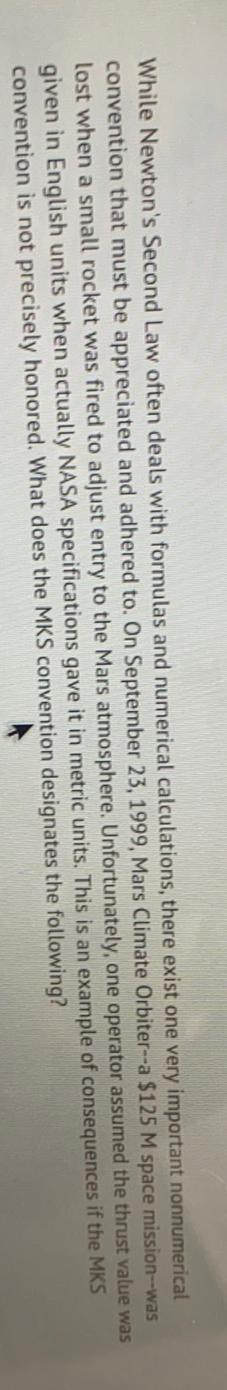Question:

While Newton's Second Law often deals with formulas and numerical calculations, there exist one very important nonnumerical convWhile Newton's Second Law often deals with formulas and numerical calculations, there exist one very important nonnumerical convention that must be appreciated and adhered to. On September 23, 1999, Mars Climate Orbiter--a \$125 M space mission--was lost when a small rocket was fired to adjust entry to the Mars atmosphere. Unfortunately, one operator assumed the thrust value was given in English units when actually NASA specifications gave it in metric units. This is an example of consequences if the MKS convention is not precisely honored. What does the MKS convention designates the following?